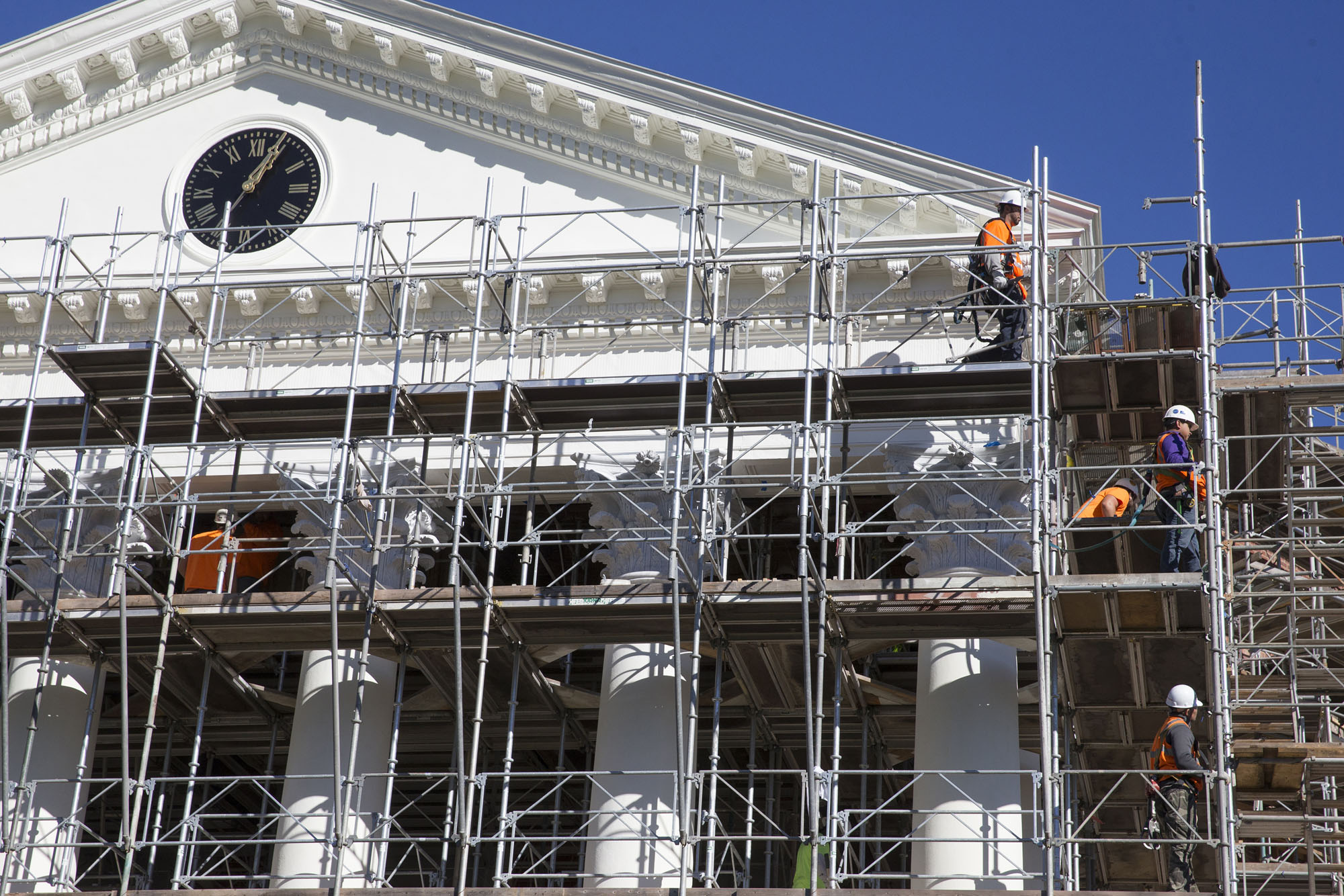Q
Scaffolding Erection Safety Standards Assessment Calculation Method
AFirst, the basic conditions

Surface roughness as Class C, the basic wind pressure Wo = 0.45KN / , Li net mesh size of 3.5cm × 3.5cm, rope diameter 3.2mm, weight 0.01KN / .

Second, the erection height calculation

Checking the site should generate bending stress analysis to determine the size, so first calculate wind loads generated pressure should be based on wind loads for δw

δw computing

Legislative Network enclosed wind factor and wind load carrier type factor “building structures norms” in the following approximate calculation method

ξ = (3.5 + 3.5) × 0.32 / (3.5 × 3.5) × 1.05 = 0.192

1.05 to consider the impact of the rope tendons

μS = 1.2 × 0.192 = 0.23

Legislative Network column passed wind load standard value (4-4) to calculate, calculate the height of the wind pressure coefficients μZ Table 4-19 in Class C values, when H = 24m time, μz = 1.25, when H = 5m, μz = 0.54 The design maximum erection height of 24m, so take u2 = 1.25.

WK = 07μzμSWO

Pressure height factor which μ2-

μS- hand aircraft carrier type wind load factor

WO- this pressure

WK = 0.7 × 1.25 × 0.23 × 0.45 = 0.09KN / 

Wind acting on the column line load standard value:

Qwk = WK? L = 0.09 × 1.8 = 0.162KN / m

Moment generating wind load calculation according to equation 4-33

MW = 1.4qWK? H2 / 10

Where Qwk– wind line load standard value

WK– vertical wind load standard value on the surface of the scaffold

L– scaffolding column spacing

Resistance moment W– column section using Table 4-31

δW– calculated wind load on the column segment generated bending stress, δW = MW / W

MW = 1.4 × 0.162 × 1.82 / 10 = 0.073KN? M

In the 24m height wind load column segment bending pressure:

δW = MW / W = (0.073 × 106) / (5.08 × 103) = 14.37N / mm2

5m height in the column section bending wind load pressure:

δW = 14.37 × (0.54 / 1.25) = 6.21N / mm2

TIANDI SCAFFOLDING & FORMWORK MANUFACTURE CO.,LTD customer perspective thinking, actively mobilizing to provide customers with safe and fast construction platform. I produced the mobile scaffold in accordance with JG13-1999 standard promulgated by the Ministry of Construction production of mobile scaffolding, 1.7 meters high, 0.95 meters wide and 1.8 meters long. Another one meter high, 0.95 meters wide and 1.8 meters long in order to meet the needs of social development, the company conducted a specialized division team, forming a complete service, high degree of specialization, the strength of the scaffolding service system.